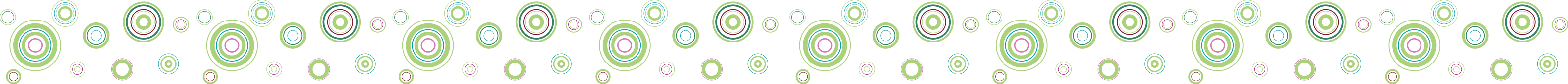Home About Us School Visits Elementary Schools Junior High Schools Senior High Schools Events at Dalhousie Virtual Classroom Visits Extra-Curricular Activities (updated) Contact Us Follow @NSMathCircles   NS Math Circles
Elementary Schools

NS Math Circles is expanding to include Elementary Schools!

The below is a list of presentations appropriate for elementary school classes, in alphabetical order. If you would like us to come and give any of these presentations in your classroom for free, please use the contact form on the School Visits page.

Candy GameLanguage: English

Four of you received four jars full of candies for being respectful to your teacher and your peers! But you find out that not all jars have the same number of candies, and you want to make sure that everyone in your group has the same number of candies. But there is a twist on how you can move candies around, and it's up to you to be fair to your peers in the most efficient way possible.

Outcomes:

• Study the mathematics behind the strategies to a game.
• Find leverages to come up with the most efficient method to solve a mathematical problem.
• Use logic to explain why you method is the optimal method.

Dots and Boxes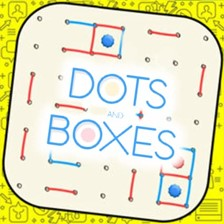Language: English

Dots and Boxes is a game invented by French mathematician Édouard Lucas, who first published a paper on it in 1895. Lucas called it la pipopipette. It is generally a two-player game, although it can be adapted to three or more players. The game is played on a grid of dots, and the goal is to form the most boxes by filling in edges between the dots. Dots and Boxes is an excellent introduction to games involving strategy. Learning this game can increase your capacity for strategic thinking, but it is also a lot of fun! We will guide you through the simple rules by going through an example, and then let you play and experiment to see what outcomes you can achieve.

Outcomes:

• Introduction to an important and deep branch of mathematics, namely Game Theory.
• Introduction to posing problems in the context of a game: i.e.: what happens if we change the rules a little bit?
• Develop strategic thinking in a fun setting.

Exploding Buckets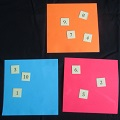We explore a classic problem of placing numbers into buckets. Be careful though: a bucket will explode if two numbers add to a third number in the same bucket (ex: 1+2 = 3 KABOOM!). How many numbers can you place into two buckets without the buckets exploding? Three buckets?

Outcomes:

• Use mental math to determine a sum to 20.
• Understand the order in which numbers are added does not affect the sum.
• Estimate the sum of two numbers to determine if it is larger than another number.

This presentation can also be done with a grade 1 class as long as your students can add up to 20.

Exploring Mathematics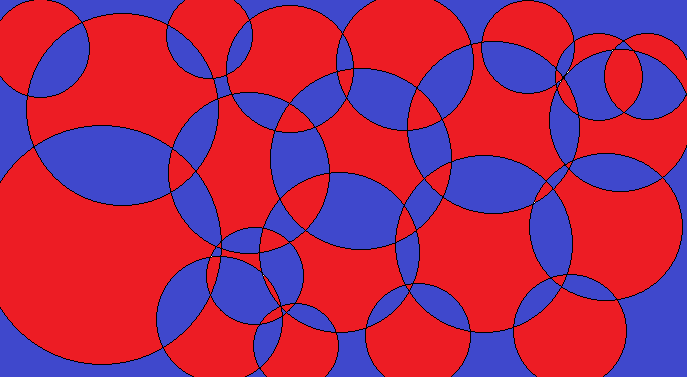Explore mathematics with a series of small problems which investigate geometry, arithmetic, map colourings and pattern recognition.

Outcomes:

• Use spatial skills to solve problems.
• Learn how to eliminate possible solutions to a problem.
• Identify if a number if even or odd.
• Finding patterns in sets of numbers.
• Use mental math to determine a sum to 20.

Fun with Fractions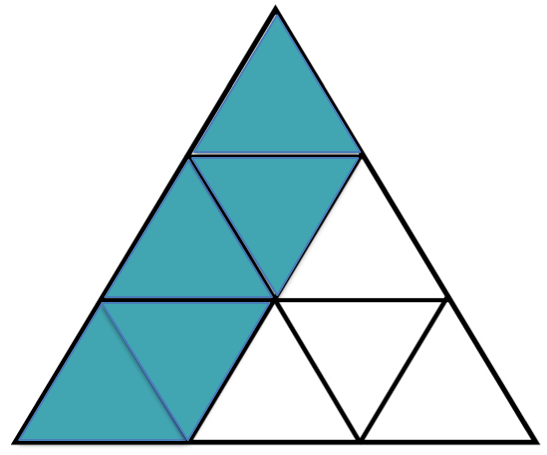Language: English

We introduce and explore the world of fractions! Using visual and real life examples as well as fraction based games we make fractions easy and fun.

Outcomes:

• Describe everyday situations where fractions are used.
• Sort a given set of diagrams of regions into those that represent equal parts and those that do not.
• Represent a given fraction concretely or pictorially.
• Name and record the fraction represented by the shaded and non-shaded parts of a given region.
• Identifying equivalent fractions (Grades 5 and 6).
• Relating improper fractions to mixed numbers and mixed numbers to improper fractions (Grade 6).

Jury Duty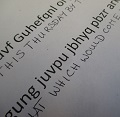Language: English

A crime has been committed! By exploring various areas of mathematics, such as logic, graph theory, cryptography and more, we will see if we can put the guilty party behind bars!

Outcomes:

• Use math to solve problems that seem to have unpredictable solutions.
• Attempt to think outside the box to solve math problems.
• Determine and explain strategies for solving problems or winning games.
• Apply logic to solve problems.
• Learn how to eliminate possible solutions to a problem.
• Introduction the mathematical areas that are currently studied: graph theory, cryptography, logic, algebra, and number theory.

MathemagicLanguage: English

Can you always correctly guess someone's birthday, magically untie yourself from ropes, or read someone's mind? You should be able to! It is not magic, it's math!

Outcomes:

• Finding patterns in sets of numbers.
• Mental math skills and applying these to concrete problems.
• Use spatial skills to solve problems.

Pascal's TriangleLanguage: English

Pascal's triangle is an array of numbers arranged in a triangular shape which, upon closer inspection, contains fascinating patterns. In this presentation we explore what information Pascal's triangle contains and discover that it has connections to combinatorics ("art of counting"), number theory, geometry and even fractals. We will also see an idea that seems true, intuitively, but is surprisingly not yet proven.

Outcomes:

• Observe what happens when you sum up numbers in certain ways, and detect any patterns.
• Think about numbers geometrically and visually, and draw the connection between numbers and geometric shapes.
• Use Pascal's triangle to solve counting problems.
• Understand what it means to "show" a claim in the world of mathematics.
• Try to pose appropriate mathematical questions to explore.

PentominoesLanguage: English

Can you always correctly guess someones birthday, magically untie yourself from ropes, or read someones mind? You should be able to! It is not magic, it's math.

Outcomes:

• Use spatial skills to solve problems.
• Select 2-D shapes from a given set of 2-D shapes to reproduce a given composite 2-D shape.

Problem Solving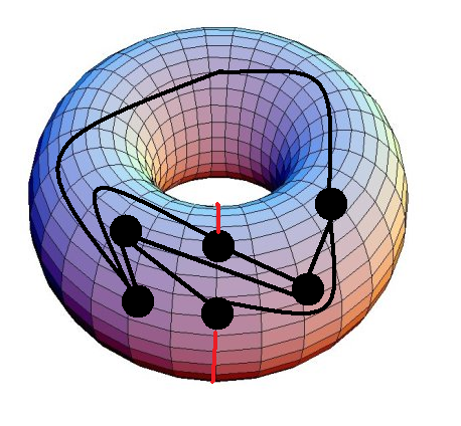Language: English

Explore the art of problem solving by investigating tiling problems, number games and graph theory problems.

Outcomes:

• Use logic to solve "hidden" math problems.
• Real life applications of math.

Tessellations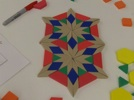Language: English

We will explore geometry in artwork and nature through tessellations. We will also play a game that illustrates the challenges of describing tessellations.

Outcomes:

• Learn to use geometry knowledge and apply it to a historical math problem.
• Learn about regular polygons. (For younger grades we simply talk about corners being same/different and try to avoid discussing angles).
• Application of math to art.

 Many Thanks to our Partners!Copyright www.nsmathcircles.com All Rights Reserved.